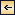Statics and Kinematics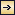### The Field of Motion and the Equation of Continuity

The Field of Motion. Among vector fields the field of motion is of special importance. Several of the characteristics of the field of motion can be dealt with without considering the forces which have brought about or which maintain the motion, and these characteristics form the subject of kinematics.

The velocity of a particle relative to a given coordinate system is defined as ν = dr/dt, where dr is an element of length in the direction in which the particle moves. In a rectangular coordinate system the velocity has the components[Equation]

The velocity field can be completely described by the Lagrange or by the Euler method. In the Lagrange method the coordinates of all moving particles are represented as functions of time and of a threefold multitude of parameters that together characterize all the moving particles. From this representation the velocity of each particle, and, thus, the velocity field, can be derived at any time.

The more convenient method by Euler will be employed in the following. This method assumes that the velocity of all particles of the fluid has been defined. On this assumption the velocity field is completely described if the components of the velocity can be represented as functions of the coordinates and of time: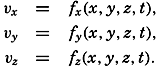[Equation]

The characteristic difference between the two methods is that Lagrange's method focuses attention on the paths taken by all individual particles, whereas Euler's method focuses attention on the velocity at each point in the coordinate space. In Euler's method it is necessary, however, to consider the motion of the individual particles in order to find the acceleration. After a time dt, a particle that, at the time t, was at the point (x,y,z) and had the velocity components fx(x,y,z,t), and so on, will be at the point (x + dx, y + dy, z + dz), and will have the velocity components fx(x + dx, y+ dy, z + dz, t + dt), and so on. Expanding in Taylor's series, one obtains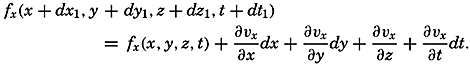[Equation]
The change in velocity in the time dt—that is, the acceleration of the
423
individual particles under consideration—will therefore have the components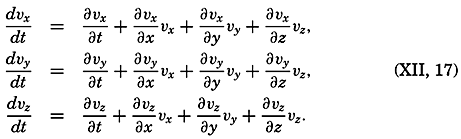[Equation]

Thus, one has to deal with two time derivatives: the individual time derivative, which represents the acceleration of the individual particles, and the local time derivative, which represents the time change of the velocity at a point in space and is called the local acceleration. The last terms in equation (XII, 17) are often combined and called the field acceleration.

The above development is applicable not only when considering the velocity field, but also when considering any field of a property that varies in space and time (p. 157). The velocity field is stationary when the local time changes are zero: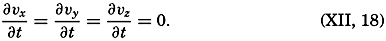[Equation]
It should be observed that, when the individual acceleration vanishes—that is, when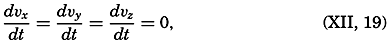[Equation]
the velocity field is stationary only if the field acceleration also disappears.

The Equation of Continuity. Consider a cube of volume dxdydz. The mass of water that in unit time flows in parallel to the x axis is equal to pvxdydz, and the mass that flows out is equal to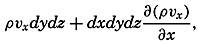[Equation]
supposing that both ρ and νx vary in the direction of the x axis. The net outflow per unit time and per unit volume in the direction of the x axis is, therefore, ∂(ρνx)/∂x Similarly, the corresponding net outflow along the y and z axes is ∂)ρνy)/∂y and ∂(ρνz)/∂z, respectively. The net outflow from a cube of unit volume is the sum of these terms, but this outflow per unit time must also equal the decrease of the density per unit time, -∂ρ/∂t. The condition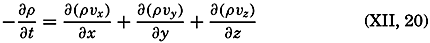[Equation]

424
must therefore always be fulfilled in order to maintain the continuity of the system. This fundamentally important equation is called the equation of continuity. It tells that the local loss of mass, represented by -∂ρ/∂t, equals the divergence of the specific momentum (p. 420).

Now: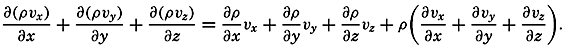[Equation]
By means of equation (XII, 17), therefore,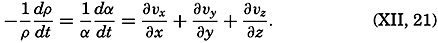[Equation]
The term on the left-hand side represents the rate of expansion of the moving element. In this form the equation of continuity states that the rate of expansion of the moving element equals the divergence of the velocity.

The equation of continuity is not valid in the above form at a boundary surface because no out- or inflow can take place there. In a direction normal to a boundary a particle in that surface must move at the same velocity as the surface itself. If the surface is rigid, no component normal to the surface exists and the velocity must be directed parallel to the surface. The condition[Equation]
where n is directed normal to the boundary surface and dn/dt is the velocity of the boundary surface in this direction, represents the kinematic boundary condition, which at the boundary takes the place of the equation of continuity.

Application of the Equation of Continuity. At the sea surface the kinematic boundary condition must be fulfilled. Designating the vertical displacement of the sea surface relative to a certain level of equilibrium by η, and taking this distance positive downward, because the positive z axis is directed downward, one obtains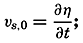[Equation]
that is, the vertical velocity at the sea surface is equal to the time change of the elevation of the sea surface. If the sea surface remains stationary, one has νz,0 = 0. If the bottom is level, one has, similarly, νz,h = 0, where h is the depth to the bottom.

With stationary distribution of mass (∂ρ/∂t = 0) the equation of continuity is reduced to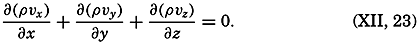[Equation]

425

The total transport of mass through a vertical surface of unit width reaching from the surface to the bottom has the components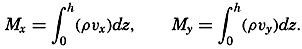[Equation]
Multiplying equation (XII, 23) by dz and integrating from the surface to the bottom, one obtains[Equation]
Here, νz,h = 0, and at stationary sea level νz,0 = 0. Thus, the equation is reduced to[Equation]
or, when the sea level remains stationary, the transport between the surface and the bottom is free of divergence.

When dealing with conditions near the surface, one can consider the density as constant and can introduce average values of the velocity components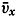[Equation] and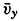[Equation] within a top layer of thickness H. With these simplifications, one obtains, putting νz,0 = 0,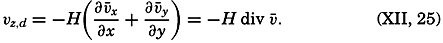[Equation]
If H is small enough, the average velocity will not differ much from the surface velocity. Since a negative vertical velocity represents an ascending motion, and a positive vertical velocity represents a descending motion, equation (XII, 25) states that at a small distance below the surface ascending motion is encountered if the surface currents are diverging, and descending if the surface currents are converging. This is an obvious conclusion, because, with diverging surface currents, water is carried away from the area of divergence and must be replaced by water that rises from some depth below the surface, and vice versa. Thus, conclusions as to vertical motion can be based on charts showing the surface currents.

For this purpose, it is of advantage to write the divergence of a two-dimensional vector field in a different form: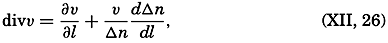[Equation]
where dl is an element of length in the direction of flow and where Δn represents the distance between neighboring stream lines. If the velocity is constant along the stream lines (∂ν/∂l = 0), the flow is divergent when the distance between the stream lines increases (dΔn/dl > 0), and convergent when the distance decreases (dΔn/dl > 0). When, on the
426
other hand, the distance between the stream lines remains constant (dΔn/dl = 0), the divergence depends only upon the change of velocity along the stream lines. Increasing velocity (∂ν/∂l > 0) means divergence accompanied by ascending motion below the surface if this surface remains stationary, and decreasing velocity (∂ν/∂l < 0) means convergence associated with descending motion below the surface.

The equation of continuity is applicable not only to the field of mass but also to the field of a dissolved substance that is not influenced by biological activity. Let the mass of the substance per unit mass of water be s. Multiplying the equation of continuity by s and integrating from the surface to bottom, one obtains, if the vertical velocity at the surface is zero,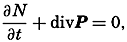[Equation]
where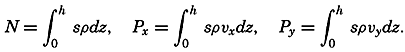[Equation]
Under stationary conditions the local time change is zero, and one has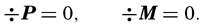[Equation]

These equations have already been used in simplified form in order to compute the relation between inflow and outflow of basins (p. 147). Other simplifications have been introduced by Knudsen, Witting, and Gehrke (Krümmel, 1911, p. 509–512).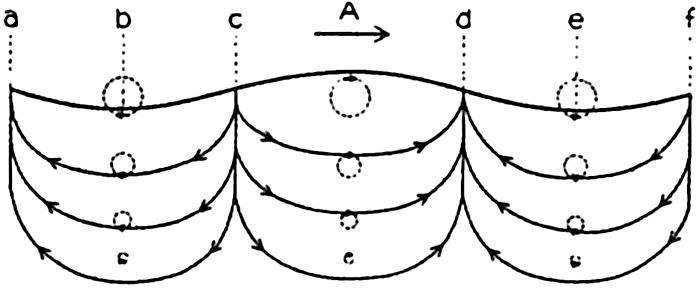#### Trajectories (full drawn lines) and stream lines (dashed lines) in a progressive surface wave.

[Full Size]

Stream Lines and Trajectories. The vector lines showing the direction of currents at a given time are called the stream lines, or the lines of flow. The paths followed by the moving water particles, on the other hand, are called the trajectories of the particles. Stream lines and trajectories are identical only when the motion is stationary, in which case the stream, lines of the velocity field remain unaltered in time, and a particle remains on the same stream line.

The general difference between stream lines and trajectories can be illustrated by considering the type of motion in a traveling surface wave. The solid lines with arrows in fig. 98 show the stream lines in a cross section of a surface wave that is supposed to move from left to right, passing the point A. When the crest of the wave passes A, the motion of the water particles at A is in the direction of progress, but with decreasing

427
velocities downward. At the troughs of the wave, at b and e, the motion is in the opposite direction. Between A and b there is, therefore, a divergence with descending motion, and between A and e there is a convergence with ascending motion. The surface will therefore sink at c and rise at d, meaning that the wave will travel from left to right. When the point c reaches A, there will be no horizontal motion of the water, but as b passes A the motion will be reversed. Thus, the pattern of stream lines moves from left to right with the velocity at which the wave proceeds.

It is supposed that the speed at which the wave travels is much greater than the velocity of the single water particles that take part in the wave motion. On this assumption a water particle that originally was located below A will never be much removed from this vertical and will return after one wave period to its original position. The trajectories of such particles in this case are circles, the diameters of which decrease with increasing distance from the surface, as shown in the figure. It is evident that the trajectories bear no similarity to the stream lines.Statics and Kinematics